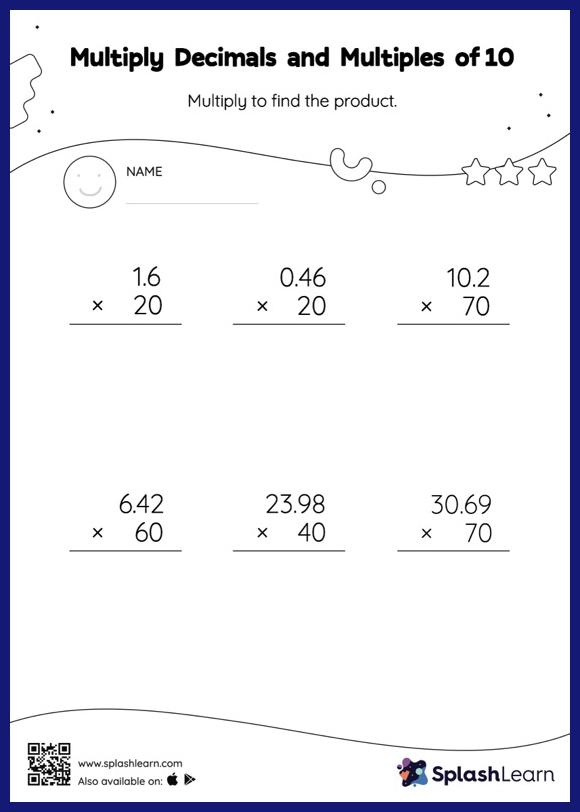# Multiply Decimals and Multiples of 10: Vertical Multiplication Worksheet

Home > Multiply Decimals and Multiples of 10: Vertical MultiplicationWhen students multiply a decimal by a number ending in zeros, they first ignore the decimal point, multiply the numbers, and then add the decimal point in the product as many places from the right as the number of decimal digits in the original number. This worksheet is about practicing the column method in which numbers are written one on top of another. In this method, students use their place value understanding to solve the problems.